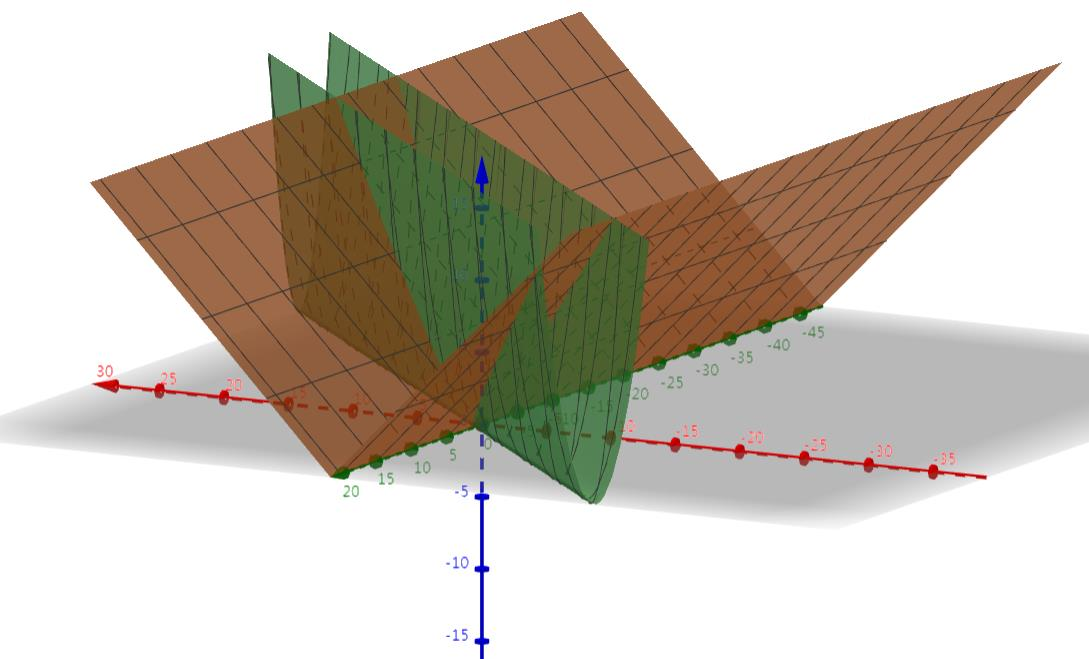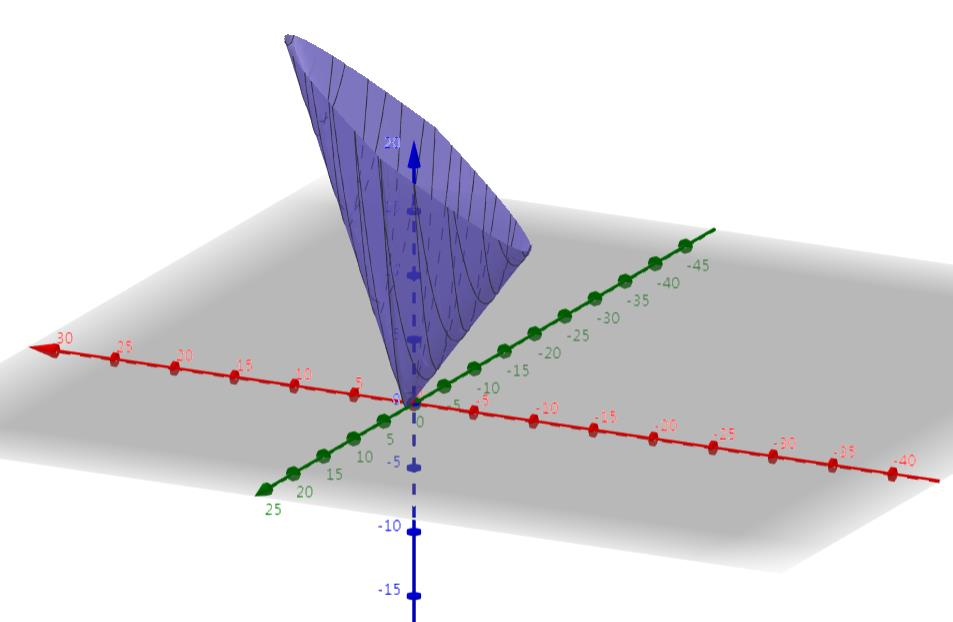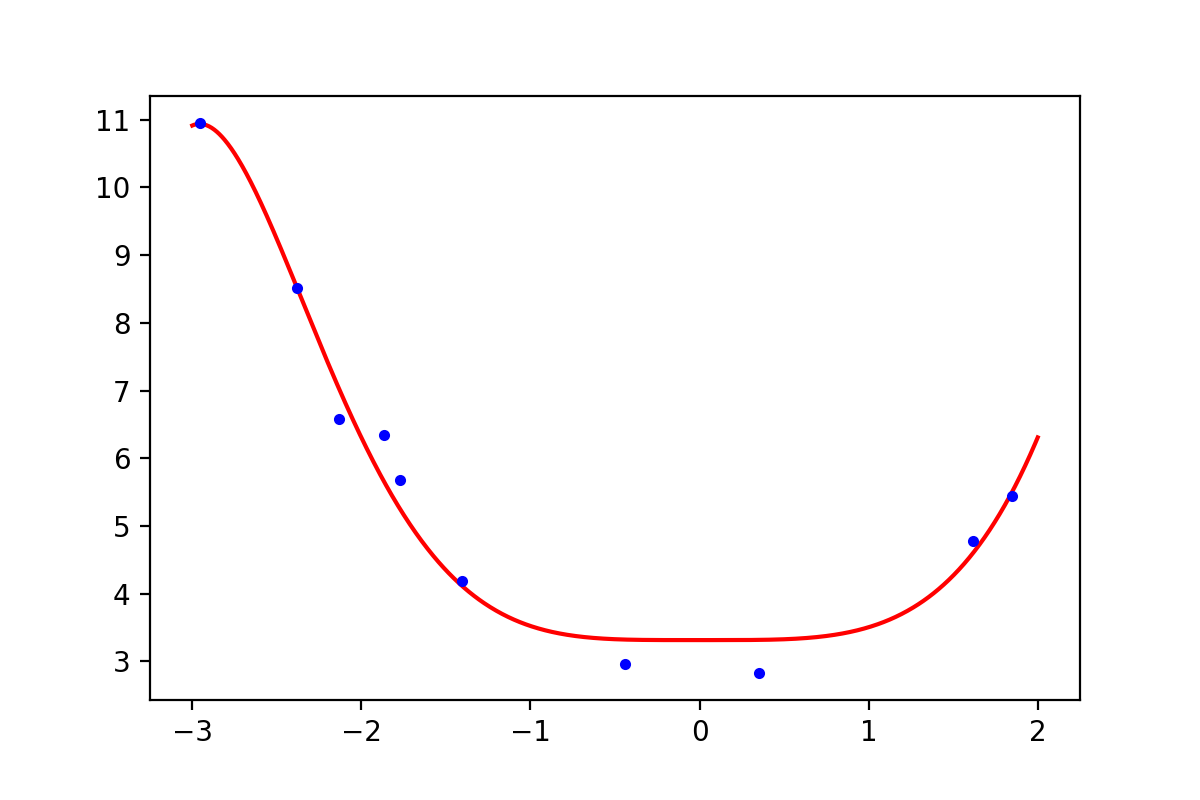【机器学习】正则化的线性回归 —— 岭回归与Lasso回归

：正则化是用来防止过拟合的方法。在最开始学习机器学习的课程时，只是觉得这个方法就像某种魔法一样非常神奇的改变了模型的参数。但是一直也无法对其基本原理有一个透彻、直观的理解。直到最近再次接触到这个概念，经过一番苦思冥想后终于有了我自己的理解。

0. 正则化（Regularization ）

• 加上所有参数（不包括$\theta_0$）的绝对值之和，即$l1$范数，此时叫做Lasso回归；
• 加上所有参数（不包括$\theta_0$）的平方和，即$l2$范数的平方，此时叫做岭回归.

0.1 代价函数的图像

$\hat{h}_{\theta} = \theta_0 + \theta_1 x$，该方程只有一个特征$x$，两个参数$\theta_0$和$\theta_1$

$J(\theta) = \frac{1}{m} \sum_{i=1}^{m}{(\theta_0 + \theta_1 x^{(i)} - y^{(i)})^2}$，该代价函数为均方误差函数（MSE），其中$m$表示样本量.• 使用$J$轴表示蓝色轴线，上方为正向；
• 使用$\theta_1$表示红色轴线，左边为正向；
• 使用$\theta_0$表示绿色轴线，指向屏幕外的方向为正向.

0.2 正则化项的图像

$J(\theta) = \frac{1}{m} \sum_{i=1}^{m}{(\theta_0 + \theta_1 x^{(i)} - y^{(i)})^2} + \lambda ||\theta_1||_1$,0.3 代价函数与正则化项图像的叠加• 加上正则化项之后，此时损失函数就分成了两部分：第1项为原来的MSE函数，第2项为正则化项，最终的结果是这两部分的线性组合;
• 在第1项的值非常小但在第2项的值非常大的区域，这些值会受到正则化项的巨大影响，从而使得这些区域的值变的与正则化项近似：例如原来的损失函数沿$\theta_0 = -\theta_1$，$J$轴方向上的值始终为0，但是加入正则化项$J = |\theta_1|$后，该直线上原来为0的点，都变成了$\theta_1$的绝对值。这就像加权平均值一样，哪一项的权重越大，对最终结果产生的影响也越大;
• 如果想象一种非常极端的情况：在参数的整个定义域上，第2项的取值都远远大于第一项的取值，那么最终的损失函数几乎100%都会由第2项决定，也就是整个代价函数的图像会非常类似于$J=|\theta_1|$（图0-2）而不是原来的MSE函数的图像（图0-1）。这时候就相当于$\lambda$的取值过大的情况，最终的全局最优解将会是坐标原点，这就是为什么在这种情况下最终得到的解全都为0.

1. 岭回归

$$J(\theta) = \frac{1}{m} \sum_{i=1}^{m}{(y^{(i)} - (w x^{(i)} + b))^2} + \lambda ||w||_2^2 = MSE(\theta) + \lambda \sum_{i = 1}^{n}{\theta_i^2} \ \quad \cdots \ (1 - 1)$$

$$J(\theta) = \frac{1}{2m} \sum_{i=1}^{m}{(y^{(i)} - (w x^{(i)} + b))^2} + \frac{\lambda}{2} ||w||_2^2 = \frac{1}{2}MSE(\theta) + \frac{\lambda}{2} \sum_{i = 1}^{n}{\theta_i^2} \ \quad \cdots \ (1 - 2)$$

$$\theta = (X^T X + \lambda I)^{-1}(X^T y)$$

$$\nabla_{\theta} J(\theta) = \frac{1}{m} X^T \cdot (X \cdot \theta - y) + \lambda w \ \quad \cdots \ (1 - 3)$$

$$\theta = \theta - (\frac{\alpha}{m} X^T \cdot (X \cdot \theta - y) + \lambda w) \ \quad \cdots \ (1 - 4)$$

1.1 数据以及相关函数

1 import numpy as np
2 import matplotlib.pyplot as plt
3 from sklearn.preprocessing import PolynomialFeatures
4 from sklearn.metrics import mean_squared_error
5
6 data = np.array([[ -2.95507616,  10.94533252],
7        [ -0.44226119,   2.96705822],
8        [ -2.13294087,   6.57336839],
9        [  1.84990823,   5.44244467],
10        [  0.35139795,   2.83533936],
11        [ -1.77443098,   5.6800407 ],
12        [ -1.8657203 ,   6.34470814],
13        [  1.61526823,   4.77833358],
14        [ -2.38043687,   8.51887713],
15        [ -1.40513866,   4.18262786]])
16 m = data.shape  # 样本大小
17 X = data[:, 0].reshape(-1, 1)  # 将array转换成矩阵
18 y = data[:, 1].reshape(-1, 1)

1.2 岭回归的手动实现

有了上面的理论基础，就可以自己实现岭回归了，下面是Python代码：

1 # 代价函数
2 def L_theta(theta, X_x0, y, lamb):
3     """
4     lamb: lambda, the parameter of regularization
5     theta: (n+1)·1 matrix, contains the parameter of x0=1
6     X_x0: m·(n+1) matrix, plus x0
7     """
8     h = np.dot(X_x0, theta)  # np.dot 表示矩阵乘法
9     theta_without_t0 = theta[1:]
10     L_theta = 0.5 * mean_squared_error(h, y) + 0.5 * lamb * np.sum(np.square(theta_without_t0))
11     return L_theta
12
13 # 梯度下降
14 def GD(lamb, X_x0, theta, y, alpha):
15     """
16     lamb: lambda, the parameter of regularization
17     alpha: learning rate
18     X_x0: m·(n+1), plus x0
19     theta: (n+1)·1 matrix, contains the parameter of x0=1
20     """
21     for i in range(T):
22         h = np.dot(X_x0, theta)
23         theta_with_t0_0 = np.r_[np.zeros([1, 1]), theta[1:]]  # set theta = 0
24         theta -= (alpha * 1/m * np.dot(X_x0.T, h - y) + lamb*(theta_with_t0_0))  # add the gradient of regularization term
25         if i%50000==0:
26             print(L_theta(theta, X_x0, y, lamb))
27     return theta
28
29 T = 1200000  # 迭代次数
30 degree = 11
31 theta = np.ones((degree + 1, 1))  # 参数的初始化，degree = 11，一个12个参数
32 alpha = 0.0000000006 # 学习率
33 # alpha = 0.003  # 学习率
34 lamb = 0.0001
35 # lamb = 0
36 poly_features_d = PolynomialFeatures(degree=degree, include_bias=False)
37 X_poly_d = poly_features_d.fit_transform(X)
38 X_x0 = np.c_[np.ones((m, 1)), X_poly_d]  # ADD X0 = 1 to each instance
39 theta = GD(lamb=lamb, X_x0=X_x0, theta=theta, y=y, alpha=alpha)

[[  1.00078848e+00]
[ -1.03862735e-05]
[  3.85144400e-05]
[ -3.77233288e-05]
[  1.28959318e-04]
[ -1.42449160e-04]
[  4.42760996e-04]
[ -5.11518471e-04]
[  1.42533716e-03]
[ -1.40265037e-03]
[  3.13638870e-03]
[  1.21862016e-03]]
3.59934190413

1 X_plot = np.linspace(-2.99, 1.9, 1000).reshape(-1, 1)
2 poly_features_d_with_bias = PolynomialFeatures(degree=degree, include_bias=True)
3 X_plot_poly = poly_features_d_with_bias.fit_transform(X_plot)
4 y_plot = np.dot(X_plot_poly, theta)
5 plt.plot(X_plot, y_plot, 'r-')
6 plt.plot(X, y, 'b.')
7 plt.xlabel('x')
8 plt.ylabel('y')
9 plt.show()图1-1，手动实现岭回归的效果

1.2 正规方程

其中$\lambda = 10$

1 theta2 = np.linalg.inv(np.dot(X_x0.T, X_x0) + 10*np.identity(X_x0.shape)).dot(X_x0.T).dot(y)
2 print(theta2)
3 print(L_theta(theta2, X_x0, y, lamb))
4
5 X_plot = np.linspace(-3, 2, 1000).reshape(-1, 1)
6 poly_features_d_with_bias = PolynomialFeatures(degree=degree, include_bias=True)
7 X_plot_poly = poly_features_d_with_bias.fit_transform(X_plot)
8 y_plot = np.dot(X_plot_poly, theta2)
9 plt.plot(X_plot, y_plot, 'r-')
10 plt.plot(X, y, 'b.')
11 plt.xlabel('x')
12 plt.ylabel('y')
13 plt.show()

[[ 0.56502653]
[-0.12459546]
[ 0.26772443]
[-0.15642405]
[ 0.29249514]
[-0.10084392]
[ 0.22791769]
[ 0.1648667 ]
[-0.05686718]
[-0.03906615]
[-0.00111673]
[ 0.00101724]]
0.604428719639图1-2，使用正规方程求解

1.3 使用scikit-learn

scikit-learn中有专门计算岭回归的函数，而且效果要比上面的方法好。使用scikit-learn中的岭回归，只需要输入以下参数：

• alpha: 上面公式中的$\lambda$，正则化项的系数；
• solver: 求解方法；
• X: 训练样本；
• y: 训练样本的标签.
1 from sklearn.linear_model import Ridge
2
3 # 代价函数
4 def L_theta_new(intercept, coef, X, y, lamb):
5     """
6     lamb: lambda, the parameter of regularization
7     theta: (n+1)·1 matrix, contains the parameter of x0=1
8     X_x0: m·(n+1) matrix, plus x0
9     """
10     h = np.dot(X, coef) + intercept  # np.dot 表示矩阵乘法
11     L_theta = 0.5 * mean_squared_error(h, y) + 0.5 * lamb * np.sum(np.square(coef))
12     return L_theta
13
14 lamb = 10
15 ridge_reg = Ridge(alpha=lamb, solver="cholesky")
16 ridge_reg.fit(X_poly_d, y)
17 print(ridge_reg.intercept_, ridge_reg.coef_)
18 print(L_theta_new(intercept=ridge_reg.intercept_, coef=ridge_reg.coef_.T, X=X_poly_d, y=y, lamb=lamb))
19
20 X_plot = np.linspace(-3, 2, 1000).reshape(-1, 1)
21 X_plot_poly = poly_features_d.fit_transform(X_plot)
22 h = np.dot(X_plot_poly, ridge_reg.coef_.T) + ridge_reg.intercept_
23 plt.plot(X_plot, h, 'r-')
24 plt.plot(X, y, 'b.')
25 plt.show()

[ 3.03698398] [[ -2.95619849e-02   6.09137803e-02  -4.93919290e-02   1.10593684e-01
-4.65660197e-02   1.06387336e-01   5.14340826e-02  -2.29460359e-02
-1.12705709e-02  -1.73925386e-05   2.79198986e-04]]
0.2138772324882. Lasso回归

Lasso回归于岭回归非常相似，它们的差别在于使用了不同的正则化项。最终都实现了约束参数从而防止过拟合的效果。但是Lasso之所以重要，还有另一个原因是：Lasso能够将一些作用比较小的特征的参数训练为0，从而获得稀疏解。也就是说用这种方法，在训练模型的过程中实现了降维(特征筛选)的目的。

Lasso回归的代价函数为：

$$J(\theta) = \frac{1}{2m} \sum_{i=1}^{m}{(y^{(i)} - (w x^{(i)} + b))^2} + \lambda ||w||_1 = \frac{1}{2}MSE(\theta) + \lambda \sum_{i = 1}^{n}{|\theta_i|} \ \quad \cdots \ (2 - 1)$$

$||w||_1$表示参数$w$的$l1$范数，也是一种表示距离的函数。加入$w$表示3维空间中的一个点$(x, y, z)$，那么$||w||_1 = |x| + |y| + |z|$，即各个方向上的绝对值（长度）之和。

$$\nabla_{\theta}MSE(\theta) + \lambda \begin{pmatrix} sign(\theta_1) \\ sign(\theta_2) \\ \vdots \\ sign(\theta_n) \end{pmatrix} \quad \cdots \ (2-2)$$

其中$sign(\theta_i)$由$\theta_i$的符号决定: $\theta_i > 0, sign(\theta_i) = 1; \ \theta_i = 0, sign(\theta_i) = 0; \ \theta_i < 0, sign(\theta_i) = -1$.

2.1 Lasso的实现

1 from sklearn.linear_model import Lasso
2
3 lamb = 0.025
4 lasso_reg = Lasso(alpha=lamb)
5 lasso_reg.fit(X_poly_d, y)
6 print(lasso_reg.intercept_, lasso_reg.coef_)
7 print(L_theta_new(intercept=lasso_reg.intercept_, coef=lasso_reg.coef_.T, X=X_poly_d, y=y, lamb=lamb))
8
9 X_plot = np.linspace(-3, 2, 1000).reshape(-1, 1)
10 X_plot_poly = poly_features_d.fit_transform(X_plot)
11 h = np.dot(X_plot_poly, lasso_reg.coef_.T) + lasso_reg.intercept_
12 plt.plot(X_plot, h, 'r-')
13 plt.plot(X, y, 'b.')
14 plt.show()

[ 2.86435179] [ -0.00000000e+00   5.29099723e-01  -3.61182017e-02   9.75614738e-02
1.61971116e-03  -3.42711766e-03   2.78782527e-04  -1.63421713e-04
-5.64291215e-06  -1.38933655e-05   1.02036898e-06]
0.04512910967733. 弹性网络（ Elastic Net）

$$J(\theta) = \frac{1}{2}MSE(\theta) + r\lambda \sum_{i = 1}^{n}{|\theta_i|} + \frac{1-r}{2} \lambda \sum_{i=1}^{n} {\theta_i^2} \ \quad \cdots \ (3 - 1)$$

1 from sklearn.linear_model import ElasticNet
2
3 # 代价函数
4 def L_theta_ee(intercept, coef, X, y, lamb, r):
5     """
6     lamb: lambda, the parameter of regularization
7     theta: (n+1)·1 matrix, contains the parameter of x0=1
8     X_x0: m·(n+1) matrix, plus x0
9     """
10     h = np.dot(X, coef) + intercept  # np.dot 表示矩阵乘法
11     L_theta = 0.5 * mean_squared_error(h, y) + r * lamb * np.sum(np.abs(coef)) + 0.5 * (1-r) * lamb * np.sum(np.square(coef))
12     return L_theta
13
14 elastic_net = ElasticNet(alpha=0.5, l1_ratio=0.8)
15 elastic_net.fit(X_poly_d, y)
16 print(elastic_net.intercept_, elastic_net.coef_)
17 print(L_theta_ee(intercept=elastic_net.intercept_, coef=elastic_net.coef_.T, X=X_poly_d, y=y, lamb=0.1, r=0.8))
18
19 X_plot = np.linspace(-3, 2, 1000).reshape(-1, 1)
20 X_plot_poly = poly_features_d.fit_transform(X_plot)
21 h = np.dot(X_plot_poly, elastic_net.coef_.T) + elastic_net.intercept_
22 plt.plot(X_plot, h, 'r-')
23 plt.plot(X, y, 'b.')
24 plt.show()

[ 3.31466833] [ -0.00000000e+00   0.00000000e+00  -0.00000000e+00   1.99874040e-01
-1.21830209e-02   2.58040545e-04   3.01117857e-03  -8.54952421e-04
4.35227606e-05  -2.84995639e-06  -8.36248799e-06]
0.08077384471924. 正则化项的使用以及l1与l2的比较

1. 创建一个$\lambda$值的列表，例如$\lambda \in {0, 0.01, 0.02, 0.04, 0.08, 0.16, 0.32, 0.64, 1.28, 2.56, 5.12, 10.24}$;
2. 创建不同degree的模型（或改变其他变量）;
3. 遍历不同的模型和不同的$\lambda$值;
4. 使用学习到的参数$\theta$（包含正则化项）计算验证集上的误差（计算误差时不包含正则化项），$J_{CV}(\theta)$;
5. 选择在验证集上误差最小的参数组合（degree和$\lambda$）;
6. 使用选出来的参数和$\lambda$在测试集上测试，计算$J_{test}(\theta)$.图4-1，Lasso与岭回归的比较（俯瞰图）

7/14, 2020: 经评论区@小鸡快跑learning指出，岭回归中加在代价函数后面的各项平方和是l2范数的平方，已更正

Reference

http://scikit-learn.org/stable/modules/generated/sklearn.linear_model.Ridge.html

Géron A. Hands-on machine learning with Scikit-Learn and TensorFlow: concepts, tools, and techniques to build intelligent systems[M]. " O'Reilly Media, Inc.", 2017. github

https://www.coursera.org/learn/machine-learning

edx: UCSanDiegoX - DSE220x Machine Learning Fundamentals

posted @ 2018-03-16 21:12  昕-2008  阅读(67739)  评论(5编辑  收藏  举报